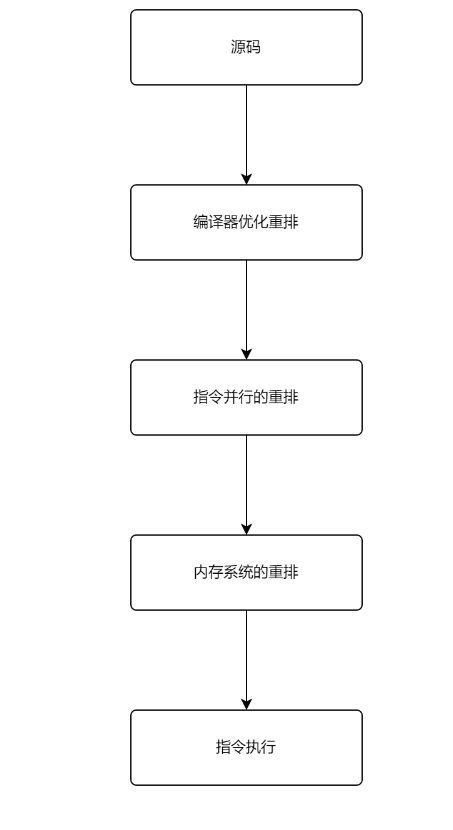# Volatile关键字能保证原子性么？

fatherlaw

0收藏

volatile
volatile 是 Java 中的一个相对来说比较重要的关键字，主要就是用来修饰会被不同线程访问和修改的变量。

volatile 又是如何保证有序性的呢？``````public class ReSortDemo {

int a = 0;
boolean flag = false;

public void mehtod1(){
a = 1;
flag = true;
}

public void method2(){
if(flag){
a = a +1;
System.out.println("最后的值: "+a);
}
}
}``````

``````class Singleton {
// 不是一个原子性操作
//private static Singleton instance;
//改进，Volatile 可以保持可见性，不能保证原子性，由于内存屏障，可以保证避免指令重排的现象产生！
private static volatile Singleton instance;

// 构造器私有化
private Singleton() {
}

// 提供一个静态的公有方法，加入双重检查代码，解决线程安全问题, 同时解决懒加载问题，同时保证了效率, 推荐使用
public static Singleton getInstance() {
if (instance == null) {
synchronized (Singleton.class) {
if (instance == null) {
instance = new Singleton();
}
}
}
return instance;
}
}``````

``````public class Test {

private static boolean flag = false;

public static void main(String[] args) throws Exception{
@Override
public void run() {
System.out.println("线程A开始执行:");
for (;;){
if (flag){
System.out.println("跳出循环");
break;
}
}
}
}).start();

@Override
public void run() {
System.out.println("线程B开始执行");
flag = true;
System.out.println("标识已经变更");
}
}).start();
}

}``````

``````线程A开始执行:``````public class Test {

private static volatile boolean flag = false;

public static void main(String[] args) throws Exception{
@Override
public void run() {
System.out.println("线程A开始执行:");
for (;;){
if (flag){
System.out.println("跳出循环");
break;
}
}
}
}).start();

@Override
public void run() {
System.out.println("线程B开始执行");
flag = true;
System.out.println("标识已经变更");
}
}).start();
}````````````public class Test {

// volatile不保证原子性
// 原子性：保证数据一致性、完整性
volatile int number = 0;

number++;
}

public static void main(String[] args) {
Test volatileAtomDemo = new Test();
for (int j = 0; j < 20; j++) {
for (int i = 0; i < 1000; i++) {
}
}, String.valueOf(j)).start();
}// 后台默认两个线程：一个是main线程，一个是gc线程
}
// 如果volatile保证原子性的话，最终的结果应该是20000 // 但是每次程序执行结果都不等于20000
" final number result = " + volatileAtomDemo.number);
}

}``````

``````main final number result = 17114
main final number result = 20000
main final number result = 19317``````

number++被拆分成3个指令

•  执行GETFIELD拿到主内存中的原始值number
•  执行PUTFIELD把工作内存中的值写回主内存中